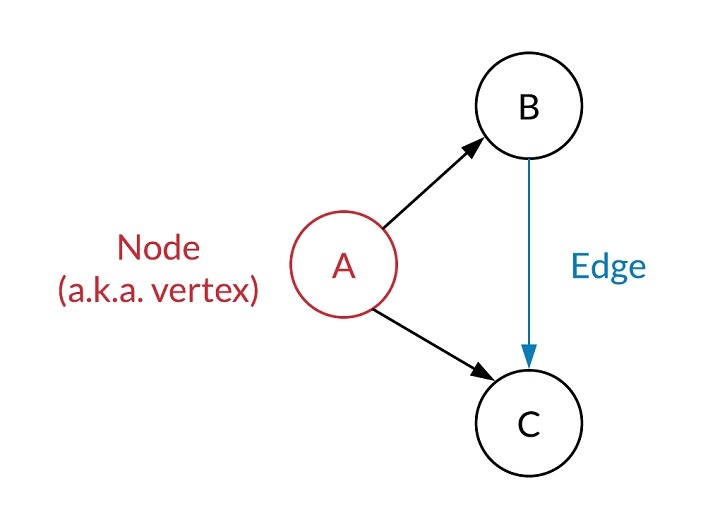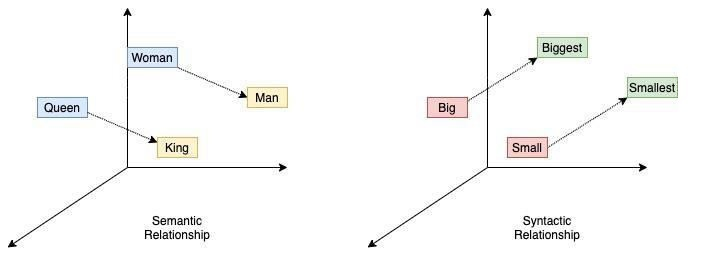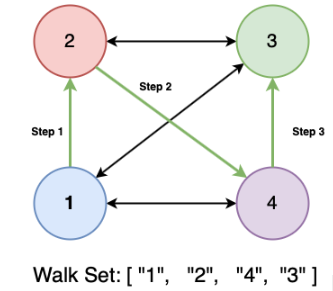# DeepWalk Algorithm

## Introduction

The graph is a very useful data structure that can represent co-interactions. These co-interactions can be encoded by neural networks as embeddings to be used in different ML Algorithms. This is where the DeepWalk algorithm shines.

In this article, we are going to explore the DeepWalk algorithm with a Word2Vec example.

## The Graph

If we consider a particular ecosystem, a graph generally represents the interaction between two or more entities. A Graph Network has two objects – node or vertex and edge.A node(or vertex) represents each element within the graph system. For example, social networks like Facebook uses Graph networks to represent users as nodes. This algorithm is used for suggesting friends.

Another aspect of the graph is the edges that connect two nodes and run from one node to another. These edges can be one-way (directional) or both ways (non-directional). It defines the interaction of the nodes. It can weight to represent the strength of interaction or link. For example, in the case of Facebook Friends suggestion algorithms discussed above, the edges connect two or more users.

Now, that we have a basic understanding of graph networks let's dive into the DeepWalk algorithm.

## The DeepWalk Algorithm

The word2Vec model allows words to be embedded into n-dimensional space vectors.Word2Vec was released by Google in 2013. In Works2Vec the words which are simar are placed close to each other in the n-dimensional space. In other words, they have the same cosine distance.The Word2Vec is trained using the skip-gram algorithm which is based on the sliding window approach. Given a particular word in the model, the skip-gram model tries to predict the surrounding words. In our scenario, we are going to predict neighbor nodes given a target node. Surrounding nodes will be encoded to find the nodes nearest to the target node.

## DeepWalk

A network's latent patterns can be identified by DeepWalk by randomly traversing graphs. Neural networks then encode and learn these patterns to produce final embeddings. An extremely simple method of generating these random paths is by starting with the target root and selecting a neighbor of that node at random, then adding it to the path at random, then choosing a neighbor of that node at random, and continuing the walk until you have taken the desired amount of steps. In the Facebook friend recommendation example, ids are generated by repeated traversing of the paths of the network. These ids are treated as tokens in a seated as in the case of the Word2Vec model.

Steps of DeepWalk Algorithm.

• Starting from a particular node, 'K-random' steps are performed from each node.

• Assign each traverse as a node and id sequence

• Using a given list of node/id strings, q Word2Vec mode is trained using the Skip-Gram algorithm.## Python code for DeepWalk algorithm

## Deepwalk
import numpy as np
import random
from tqdm import tqdm
import warnings
warnings.filterwarnings('ignore')
from sklearn.decomposition import PCA
import networkx as nx
import matplotlib.pyplot as plt
import pandas as pd
from gensim.models import Word2Vec
%matplotlib inline

df_data = pd.read_csv("/content/space_data.tsv", sep = "\t")
g = nx.from_pandas_edgelist(df_data, "source", "target", edge_attr=True, create_using=nx.Graph())
def get_random_walk(node, pathlength):

random_walk = [node]

for i in range(pathlength-1):
tmp = list(g.neighbors(node))
tmp = list(set(tmp) - set(random_walk))
if len(tmp) == 0:
break
ran_node = random.choice(tmp)
random_walk.append(ran_node)
node = ran_node
return random_walk
allnodes = list(g.nodes())

walks_list_random = []
for n in tqdm(allnodes):
for i in range(5):
walks_list_random.append(get_random_walk(n,10))
# count of sequences
len(walks_list_random)
model = Word2Vec(window = 4, sg = 1, hs = 0,
negative = 9,
alpha=0.04, min_alpha=0.0005,seed = 20)
model.build_vocab(walks_list_random, progress_per=2)

model.train(walks_list_random, total_examples = model.corpus_count, epochs=20, report_delay=1)
model.similar_by_word('artificial intelligence')


## Output

100%|██████████| 2088/2088 [00:00<00:00, 6771.58it/s]
[('robot ethics', 0.9894747138023376),
('cognitive robotics', 0.9886192083358765),
('evolutionary robotics', 0.9876964092254639),
('multi-agent system', 0.9861799478530884),
('cloud robotics', 0.9842559099197388),
('fog robotics', 0.9835143089294434),
('glossary of robotics', 0.9817663431167603),
('soft robotics', 0.9738423228263855),
('robotic governance', 0.9687554240226746),
('robot rights', 0.9686211943626404)]


Explanation - The output lists the most similar words to the search word(query) “'artificial intelligence'”.The output is a list of tuples where in each tuple the first element is a similar word and the second element is the confidence value. For eg. ‘robot ethics’ is 98.9% similar ( confidence - 0.989), then cognitive robotics(0.988) and so on

## Conclusion

DeepWalk is a highly versatile algorithm. With little tweaks, it can be implemented in many kinds of networks. It is one of the most efficient algorithms which could be considered.

Updated on: 23-Mar-2023

290 Views